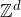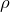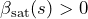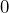# Yacine Aoun, Sébastien Ott: ON THE TWO-POINT FUNCTION OF THE POTTS MODEL IN THE SATURATION REGIME

Abstract:

We will consider FK percolation onwith infinite range interactions of the formwhereis a norm onandis a subexponential correction. We will expose optimal conditions for the existence of a ‘saturation regime’ (that is, the existence ofsuch that the inverse correlation length in the direction s is constant on [0,. Under suitable conditions, we will then explain how to derive sharp asymptotics of the two-point connectivity function (that are not of the Ornstein-Zernike type) and estimates on the volume of the connected component ofin the whole saturation regime. Our analysis uses the OSSS inequality adapted for the infinite range interactions and a one step renormalization argument to study the two-point connectivity function. Our analysis also applies in the case where the interactions decay subexponentially fast in the whole subcritical regime. The first half of the talk will be focused on exposing the results, heuristics, and remaining open problems, while the second half will be an exposition of the core ideas used in the proof.

______________________

Based on

https://arxiv.org/pdf/2207.02193.pdf

## Video